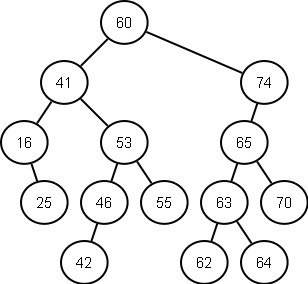## Monday, June 17, 2013

### Shortest Path in a Binary Search Tree

The shortest path in a binary search tree is not much more different than the shortest path in a binary tree that I handled here. The difference consists in reusing the binary search tree property where the left branch nodes are less than the root, and the right branch nodes are larger than the root. As in the binary tree shortest path, the procedure consists of finding the lowest common ancestor which I described here and then "drawing" the path from the ancestor to the nodes. Let's use the below tree as an example.Fig. 1 - Simple Binary Search Tree

Let's say we want to find the smallest path from "25" to "46". It is obvious that it has to go through the lowest common ancestor that we identify as "41". Now we have 2 basic cases: either one of the nodes we're looking for is the LCA and the other is in either of the branches; or each of the nodes is in a separate branch. Now if we don't have the first case, we can look for the minimum of the two nodes in the left branch and for the maximum in the right branch. And again, this is because of the binary search tree property - that the lower element goes on the left and higher on the right. So we go down on the left binary searching for our node "25" and same with "46". I say binary searching because at each step we compare the current node with the node we're looking for and decide whether to go to the right or to the left. Managing the path is tricky, since the path for the second element will come inverted with recursion. In my implementation I used a stack to manage that as I did when looking for the shortest path in a simple binary tree:

Note:
- First stack is not really needed, a simple list would do. I just like symmetry.
- For the lowestCommonAncestor method see here.

#### 1 comment:

1.Pavel, I implemented it in C and it is giving me only single path from lca to min(a,b) and not other path lca to max(a,b).
Please help me..
Here is link to my code on Ideone..
http://ideone.com/d7dWPv